# Illinois TAP Math: Geometry Chapter Exam

Exam Instructions:

Choose your answers to the questions and click 'Next' to see the next set of questions. You can skip questions if you would like and come back to them later with the yellow "Go To First Skipped Question" button. When you have completed the practice exam, a green submit button will appear. Click it to see your results. Good luck!

### Page 1

#### Question 1 1. The pictured shape is all of the following EXCEPT a: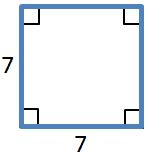#### Question 2 2. The pictured shape is a: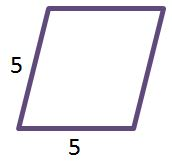#### Question 3 3. What is the perimeter of this shape?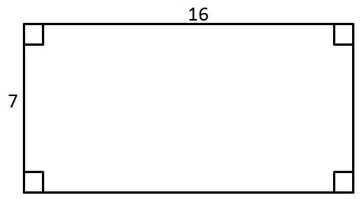#### Question 5 5. What is the relationship between Angle 1 and Angle 7?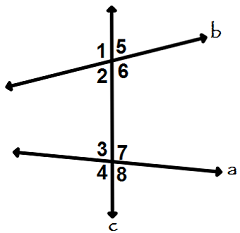### Page 2

#### Question 7 7. Given the triangle PQE, calculate the measure of Angle Q.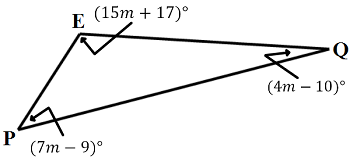#### Question 8 8. Given the figure below, Angle 3 = 140y and Angle 5 = 80y + 70. Determine the value of y.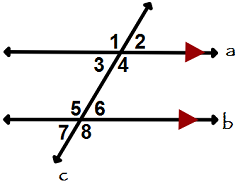#### Question 9 9. In the figure below, Angle 7 = 15x and Angle 10 = 9x + 30. Determine the measure of Angle 10.#### Question 10 10. What is the perimeter of this polygon?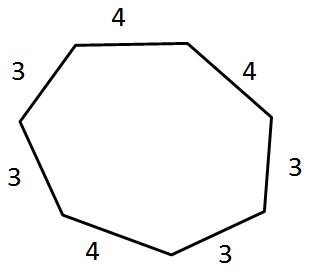### Page 3

#### Question 13 13. What is the relationship between Angle 2 and Angle 7?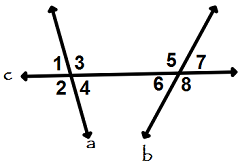#### Question 14 14. What is the area of this shape?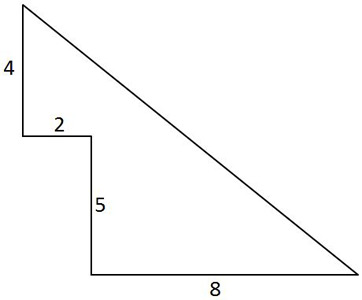#### Question 15 15. Which line is the transversal?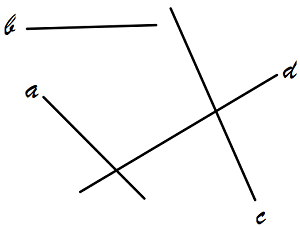### Page 4

#### Question 17 17. Which two lines are parallel?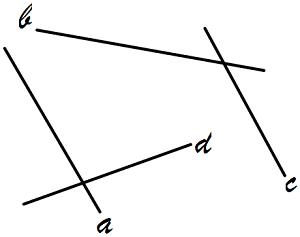#### Question 18 18. In triangle GBN, Angle G is seven more than three times a number, Angle B is five more than four times the number, and Angle N is twelve less than five times the number. Solve for the measure of Angle G.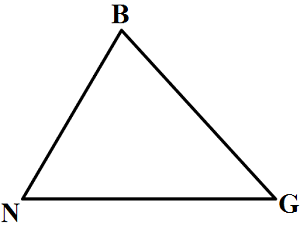#### Question 19 19. What is the area of the pictured triangle?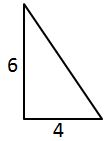### Page 5

#### Question 21 21. What is the area of this shape?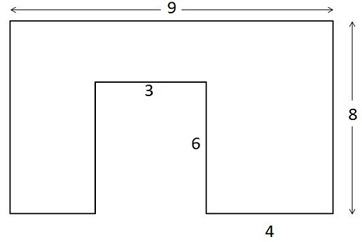#### Question 23 23. What is the area of ABCDE, as shown in the diagram?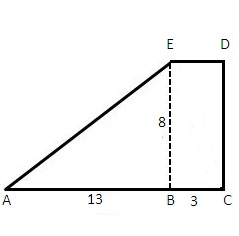#### Question 24 24. If the two triangles pictured below are similar, then what is x?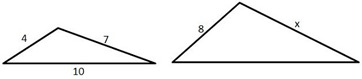### Page 6

#### Question 27 27. What is the area of this shape?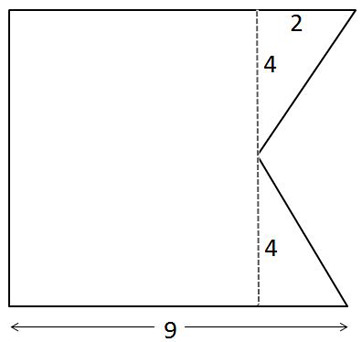#### Question 29 29. The volume of this square pyramid is 6250 cubic feet. If each side of the base is 25 feet long, what is its height?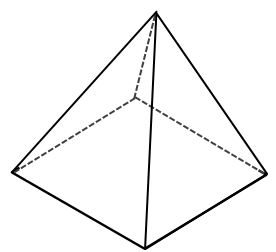#### Question 30 30. What is the relationship between Angle 4 and Angle 1?#### Illinois TAP Math: Geometry Chapter Exam Instructions

Choose your answers to the questions and click 'Next' to see the next set of questions. You can skip questions if you would like and come back to them later with the yellow "Go To First Skipped Question" button. When you have completed the practice exam, a green submit button will appear. Click it to see your results. Good luck!

Support